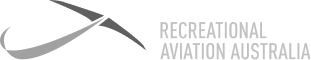# Definition: Power Sandwich

This occurs during a turn when load factor is being introduced. As the load factor increases, there is an increase in stall speed. While in a turn, drag is increased, which effectively decreases airspeed. The power sandwich occurs between the decrease in airspeed and increase in stall speed, because power needs to be added to combat the increased drag to maintain a margin over the stall speed. To calculate a new stall speed with an increased load factor, we can use √g x stall speed = new stall speed with increased load factor.### Power Sandwich

This occurs during a turn when load factor is being introduced. As the load factor increases, there is an increase in stall speed. While in a turn, drag is increased, which effectively decreases airspeed. The power sandwich occurs between the decrease in airspeed and increase in stall speed, because power needs to be added to combat the increased drag to maintain a margin over the stall speed. To calculate a new stall speed with an increased load factor, we can use √g x stall speed = new stall speed with increased load factor.

7 Day

FREE

Trial